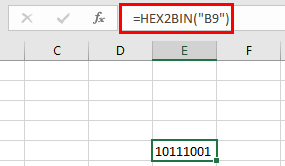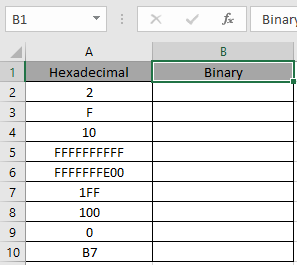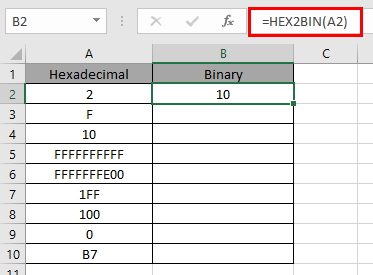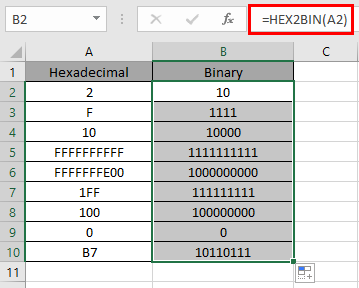# How to use the HEX2BIN Function in Excel

HEX2BIN function in excel is used to convert Hexadecimal representation of numbers of radix 16 to binary numbers (radix = 2).

The table shown below shows you some of the most used base & their radix of Alpha - numeric characters

 Base radix Alpha-Numeric Characters Binary 2 0 - 1 Octal 8 0 - 7 Decimal 10 0 - 9 hexadecimal 16 0 - 9 & A - F hexatridecimal 36 0 - 9 & A - Z

Hexadecimal number is representation of a number of radix 16. 10 digits starting from 0, 1, 2, 3, 4, 5, 6, 7, 8 ,9 along with 6 alphabets starting from A, B, C, D, E & F where Binary number is representation of a number of radix 2. Only 2 digits are used in representation of a binary number 0 & 1. The below table will help you understand better.

 Binary Decimal Hexadecimal 000 0 0 001 1 1 010 2 2 011 3 3 100 4 4 101 5 5 110 6 6 111 7 7 1000 8 8 1001 9 9 1010 10 A 1011 11 B 1100 12 C 1101 13 D 1110 14 E 1111 15 F

Conversion formulation is shown below:

Let’s take an example having 4 bits.
543

1. Separate the digits
2. Write their binary conversion upto 4 digit place by adding zeros to the left of the binary number
3. Combine all the converted binary to get the binary number.
 5 4 3 101 100 11 0101 0100 0011 543 ( Hexadecimal ) = 010101000011( binary )

The HEX2BIN function converts the Hexadecimal number of radix 16 to the binary number of radix 2.
Syntax:

=HEX2BIN ( number, [places] )

Number : Hexadecimal number which returns the 10 character binary number.
[Places] : [optional] number where result expressed upto the number.

The input value to the function cannot contain more than 10 characters ( 10 bits ). Where first number returns the sign of the number (positive or negative) & Other 9 returns the value after the sign.To get a negative number, input number must have 10 characters starting with first bit as it represents the negative sign. The negative number is calculated using two's complement notation. Any less than 10 characters, the function considers default 0s in front.

Now let’s get more understanding of the function via using them in some examples.Here we have some Hexadecimal values in Hexadecimal Column. We need to convert these Hexadecimal numbers to binary value.

Use the formula in Hexadecimal column:

=HEX2BIN (A2)

A2 : number provided to the function as cell reference or text in quotes

Values to the HEX2BIN function is provided as cell reference.The HEX2BIN representation of 2 of base 16 (hexadecimal) is 10 of base 2 (decimal).

216= 102

Now copy the formula to other cells using the Ctrl + D shortcut key.As you can see here the HEX2BIN function returns the result of the input values.

Notes:

1. Numbers can be given as argument to the function directly with quotes or cell reference in excel.
2. The function doesn’t consider the [places] number in case of a negative decimal number.
3. If the input Hexadecimal number is negative it cannot be less than FFFFFFFE00 and if the number is positive it cannot be greater than 1FF.
4. If the input [places] number is not an integer, it is truncated by the function.
5. The function returns the binary value for the max value of 111111111 & minimum value upto 1000000000 (only integers).
6. The function returns the #NUM! Error
1. If the input Hexadecimal number is less than FFFFFFFE00 or greater than 1FF.
2. If the input number [places] is zero or negative.
3. If the input number [places] is not sufficient for the resulting positive binary number.
7. The function returns the #VALUE! Error
1. If the input [places] number is text or non-numeric.

Hope you understood how to use HEX2BIN function and referring cell in Excel. Explore more articles on Excel mathematical conversion functions here. Please feel free to state your query or feedback for the above article.

Related Articles:

How to use the DEC2BIN Function in Excel

How to use the BIN2OCT Function in Excel

How to use the BIN2HEX Function in Excel

How to use the DEC2OCT Function in Excel

How to use the DEC2HEX Function in Excel

How to use the OCT2BIN Function in Excel

How to use the OCT2DEC Function in Excel

How to use the OCT2HEX Function in Excel

How to use the HEX2BIN Function in Excel

How to use the HEX2OCT Function in Excel

How to use the HEX2DEC Function in Excel

Popular Articles:

If with wildcards

Vlookup by date

Join first and last name in excel

Count cells which match either A or B

Terms and Conditions of use

The applications/code on this site are distributed as is and without warranties or liability. In no event shall the owner of the copyrights, or the authors of the applications/code be liable for any loss of profit, any problems or any damage resulting from the use or evaluation of the applications/code.